# Multicore Deployment of a Plant Model

This example illustrates how to take advantage of executing multithreaded code on a multicore processor using graphical partitioning. This example requires Simulink® Coder™ to generate multithreaded code.

### Overview

One objective of model-based design is to create realistic models of physical systems and to simulate these models in real-time, for example, to verify controllers using hardware-in-the-loop (HIL). However, as more features are added to the plant model, the computational requirements may exceed the resources available by single-core processing systems.

Partitioning the plant and controller into separate pieces is one way to address the computational needs of complex models. With Simulink® you can partition a plant using Model blocks and then assign the code generated by each sub-model to threads for real-time execution on an HIL system such as Simulink Real-Time™. To see how this works, let us use our host computer as a stand-in for the real-time execution environment and generate real-time multi-threaded code for the following model.

```slexMulticoreSolverExample ```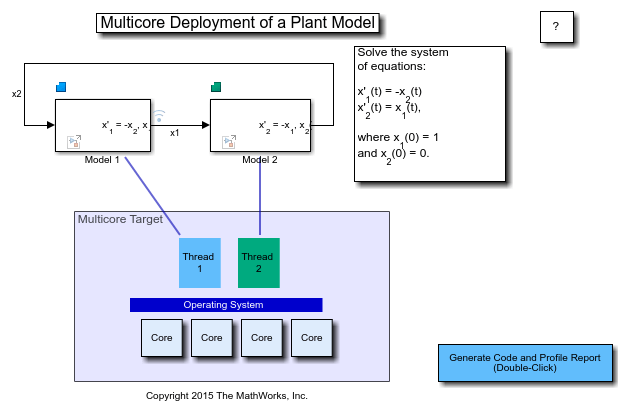### Symmetric Multicore Processing

The illustration above shows that the code generated for the model is separated into two threads. In this example, the target is assumed to be a Symmetric Multicore Processor, so that the threads are not associated with any particular core. The operating system is responsible for making the best use of the cores when scheduling thread execution. Ideally, to provide maximum flexibility, the number of threads (Nt) should be greater than the number of cores (Nc). Double-click on the 'Generate Code and Profile Report' button to generate multi-threaded code, profile its execution and visualize the results. The visualization shows a core occupancy map of how the cores were utilized at each time step of execution. It can be seen that the threads float across cores as deemed best by the operating system scheduler. This kind of scheduling is good when the operating system also needs to run other processes.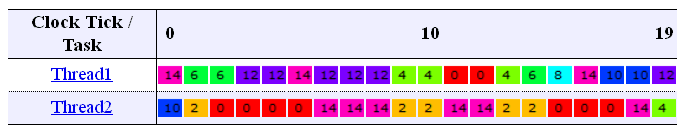Simulink® Coder™ generates code such that the two threads may execute simultaneously and possibly on two different cores. This means that the signal values for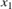and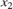must be synchronized between the two threads. Simulink® provides several options to handle this requirement as illustrated here:Using the script below, we will simulate and demonstrate the effect of the deterministic modes to understand how Simulink® handles synchronization.

• Reference solution (ode3) - Simulink® is configured to provide the reference solution by synchronizing data at each major and minor time step.

• Zero Order Hold - Each thread is solving a sub-system of equations using its own solver, while synchronizing data only on major time steps.

• Linear extrapolation - In addition to the Zero Order Hold mode, each solver extrapolates data using linear predictions to compensate for data latency errors.

For most systems where the synchronization points are smooth, the linear extrapolation mode provides a good tradeoff between communication bottlenecks and numerical accuracy.

```h = figure; hVal = ishold; hold on; mdl = 'slexMulticoreSolverExample'; dt = get_param(mdl, 'DataTransfer'); modes = { ... 'Ensure deterministic transfer (minimum delay)', ... 'None', 'k:', ... 'Ensure deterministic transfer (maximum delay)', ... 'Zero Order Hold', 'm', ... 'Ensure deterministic transfer (maximum delay)', ... 'Linear', 'b' ... }; for i=1:3:length(modes) dt.DefaultTransitionBetweenContTasks = modes{i}; dt.DefaultExtrapolationMethodBetweenContTasks = modes{i+1}; out = sim(mdl); plot(out.logsout.get('x1').Values.Time, ... out.logsout.get('x1').Values.Data, ... modes{i+2}); end legend('Reference solution (ode3)', ... 'Zero Order Hold Extrapolation', ... 'Linear Extrapolation'); ```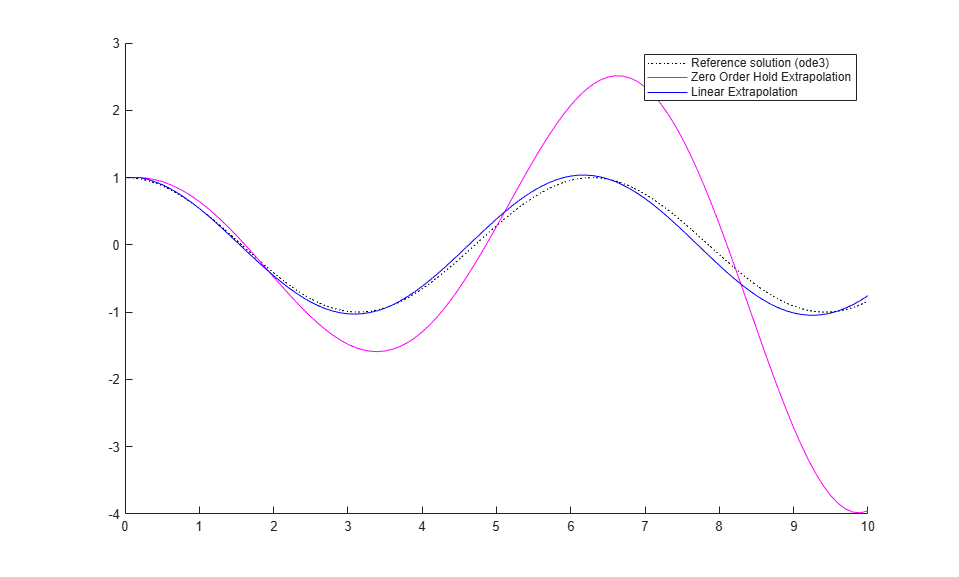### Closing the Models

```close_system('slexMulticoreSolverExample',0); close_system('slexMulticoreSolverMdlref',0); if ~hVal, hold off; end delete(h); ```